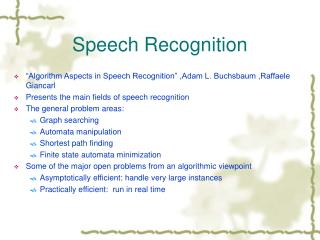DownloadDownload PresentationSpeech Recognition

# Speech Recognition

Télécharger la présentation## Speech Recognition

- - - - - - - - - - - - - - - - - - - - - - - - - - - E N D - - - - - - - - - - - - - - - - - - - - - - - - - - -
##### Presentation Transcript

1. Speech Recognition • “Algorithm Aspects in Speech Recognition” ,Adam L. Buchsbaum ,Raffaele Giancarl • Presents the main fields of speech recognition • The general problem areas: • Graph searching • Automata manipulation • Shortest path finding • Finite state automata minimization • Some of the major open problems from an algorithmic viewpoint • Asymptotically efficient: handle very large instances • Practically efficient: run in real time

2. Block diagram of speech recognizer

3. IWR , CSR • IWR: Isolated Word Recognition • Spoken in isolation and belonging to a fixed dictionary • Lexicon: typical pronunciations of each word in dictionary • Search algorithm: output the word that maximizes a given objective function ( likelihood of a word given the observation sequence) • CSR: Continuous Speech Recognition • Lexicon: same as IWR • Language model: give a stochastic description of the language and the possibly probabilistic description of which specific words can follow another word or group of words • Search algorithm: find a grammatically correct sentence that maximizes a given objective function ( likelihood of a sentence given the observation sequence) • Coarticulation effects: “how to recognize speech” vs “how to wreck a nice beach”, incomplete information

4. Major methods for speech recognition • Template-based approach • Small dictionaries, mainly for IWR • Reference templates (a sequence of feature vectors representing a unit of speech to be recognized) • Distance measure eg: log spectral distance, likelihood distortions • Stochastic approach (maximum likelihood) • Dominant method • Equations represent • X: observation sequence • W: unknown sentence • Output the sentence ŵthat Pr(ŵ) = max w{ Pr(W|X) } Pr(W|X) * Pr(X) = Pr(X|W) * Pr(W) Ŵ = argmax w { Pr(X|W) * Pr(W) } for fixed X ( argmaxw{ f(w) } = ŵ  f(ŵ) = max w{ f(w) } • Defn: Cs = -logPr eg: Cs(W) = - logPr(W) • Ŵ = argmin w{ Cs(W) + Cs(X|W) } • Solution of the equation: Language Modeling and Acoustic Modeling

5. Modeling Tools • HMM (Hidden Markov Model) • Quintuple λ= (N,M,A,B,π) • N: the number of state • M: the number of symbols that each state can output or recognize • A: N*N state transition matrix, a(i,j) = the probability of moving from state i to state j • B: observation probability distribution, bi(δ) =the probability of recognizing or generating the symbol δwhen in state i • Π: the initial state probability distribution such that Πi = the probability of being in state i at time 1. • MS (Markov Source) • E: transitions between states • V: set of states • ∑: alphabet including null symbol • One to one mapping M from E to V*∑*V • M(t) = (i, a, j) i is the predecessor state of t t output symbol a j is the successor state of t

6. Viterbi • Viterbi Algorithm: • Compute the optimal state sequence Q = (q1,…..,qT) throughλ that matches X. (that is max Pr(Q|X,λ) ) • βt (i) = probability along the highest probability that accounts for the first t observations and ends in state i • γt (i) = the state at time t-1 that led to state I at time t along that path • Initialization: • Induction: • Termination: • Backtracking:

7. Acoustic Word Models via Acoustic Phone Models • Tree representation • Static data structure • Lexicon • Over the alphabet of feature vectors

8. MS ,HMM • Circles represent states, arcs represent transitions. • Arcs are labeled f/p, denoting that the associated transition outputs phone f and occurs with probability p • For each phone f in the alphabet build a HMM • Directed graph having a minimum of four and a maximum of seven states with exactly one source, one sink, self loops and no back arcs • Gives an acoustic model describing the different ways in which one can pronounce the given phone • Technically, this HMM is a device for computing how likely it is that a given observation sequence acoustically matches the given phone

9. MS + HMM

10. Conclusion • Language Model • Pr(W) = Pr(w1….wj) = Pr(w1)Pr(w2|w1)…..Pr(wj|w1…..wj-1) • Approximation: Pr(wj|w1….wj-1) = Pr(wj|wj-k+1….w1) • 20,000 words,k=2,400 million vertices and arcs in the model • Possible solution: group the equivalence classes (how to divide?) • Heuristic approach • Layer solution • Shortest path finding • Automata machine • Redundancy problem and size reduction • Training with efficiency

11. Application • AT&T Watson Advanced Speech Application Platform http://www.att.com/aspg/blasr.html • BBN Speech Products http://www.bbn.com/speech_prods/ • DragonDictate from Dragon Systems,Inc. http://www.dragonsys.com/